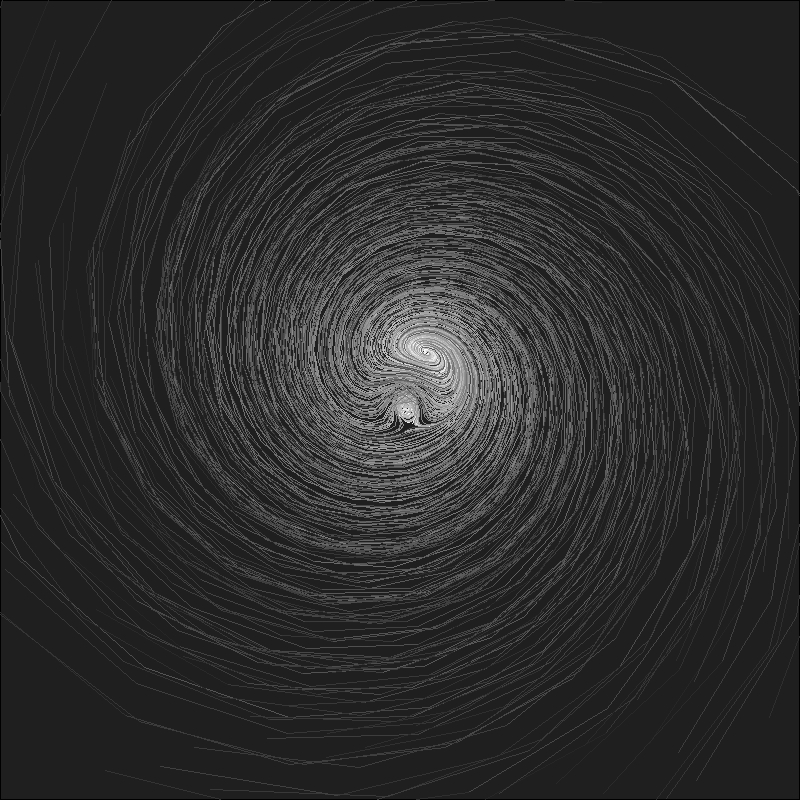# The Chaos Game: an experiment about fractals, recursivity and creative coding

Mathematics, rightly viewed, possesses not only truth, but supreme beauty (Bertrand Russell)

You have a pentagon defined by its five vertex. Now, follow these steps:

• Step 0: take a point inside the pentagon (it can be its center if you want to do it easy). Keep this point in a safe place.
• Step 1: choose a vertex randomly and take the midpoint between both of them (the vertex and the original point). Keep also this new point. Repeat Step 1 one more time.
• Step 2: compare the last two vertex that you have chosen. If they are the same, choose another with this condition: if it’s not a neighbor of the last vertex you chose, keep it. If it is a neighbor, choose another vertex randomly until you choose a not-neighbor one. Then, take the midpoint between the last point you obtained and this new vertex. Keep also this new point.
• Step 3: Repeat Step 2 a number of times and after that, do a plot with the set of points that you obtained.

If you repeat these steps 10 milion times, you will obtain this stunning image:

I love the incredible ability of maths to create beauty. More concretely, I love the fact of how repeating extremely simple operations can bring you to unexpected places. Would you expect that the image created with the initial naive algorithm would be that? I wouldn’t. Even knowing the result I cannot imagine how those simple steps can produce it.

The image generated by all the points repeat itself at different scales. This characteristic, called self-similarity, is property of fractals and make them extremely attractive. Step 2 is the key one to define the shape of the image. Apart of comparing two previous vertex as it’s defined in the algorithm above, I implemented two other versions:

• one version where the currently chosen vertex cannot be the same as the previously chosen vertex.
• another one where the currently chosen vertex cannot neighbor the previously chosen vertex if the three previously chosen vertices are the same (note that this implementation is the same as the original but comparing with three previous vertex instead two).

These images are the result of applying the three versions of the algorithm to a square, a pentagon, a hexagon and a heptagon (a row for each polygon and a column for each algorithm):

From a technical point of view I used `Rcpp`to generate the set of points. Since each iteration depends on the previous one, the loop cannot easily vectorised and C++ is a perfect option to avoid the bottleneck if you use another technique to iterate. In this case, instead of writing the C++ directly inside the `R` file with `cppFunction()`, I used a stand-alone C++ file called `chaos_funcs.cpp` to write the C++ code that I load into R using `sourceCpp()`.

Some days ago, I gave a tutorial at the coding club of the University Carlos III in Madrid where we worked with the integration of C++ and R to create beautiful images of strange attractors. The tutorial and the code we developed is here. You can also find the code of this experiment here. Enjoy!

# Chaotic Galaxies

Tell me, which side of the earth does this nose come from? Ha! (ALF)

Reading about strange attractors I came across with this book, where I discovered a way to generate two dimensional chaotic maps. The generic equation is pretty simple:$x_{n+1}= a_{1}+a_{2}x_{n}+a_{3}x_{n}^{2}+a_{4}x_{n}y_{n}+a_{5}y_{n}+a_{6}y_{n}^{2}$$y_{n+1}= a_{7}+a_{8}x_{n}+a_{9}x_{n}^{2}+a_{10}x_{n}y_{n}+a_{11}y_{n}+a_{12}y_{n}^{2}$

I used it to generate these chaotic galaxies:

Changing the vector of parameters you can obtain other galaxies. Do you want to try?

```library(ggplot2)
library(dplyr)
#Generic function
attractor = function(x, y, z)
{
c(z+z*x+z*x^2+ z*x*y+ z*y+ z*y^2,
z+z*x+z*x^2+z*x*y+z*y+z*y^2)
}
#Function to iterate the generic function over the initial point c(0,0)
galaxy= function(iter, z)
{
df=data.frame(x=0,y=0)
for (i in 2:iter) df[i,]=attractor(df[i-1, 1], df[i-1, 2], z)
df %>% rbind(data.frame(x=runif(iter/10, min(df\$x), max(df\$x)),
y=runif(iter/10, min(df\$y), max(df\$y))))-> df
return(df)
}
opt=theme(legend.position="none",
panel.background = element_rect(fill="#00000c"),
plot.background = element_rect(fill="#00000c"),
panel.grid=element_blank(),
axis.ticks=element_blank(),
axis.title=element_blank(),
axis.text=element_blank(),
plot.margin=unit(c(-0.1,-0.1,-0.1,-0.1), "cm"))
#First galaxy
z1=c(1.0, -0.1, -0.2,  1.0,  0.3,  0.6,  0.0,  0.2, -0.6, -0.4, -0.6,  0.6)
galaxy1=galaxy(iter=2400, z=z1) %>% ggplot(aes(x,y))+
geom_point(shape= 8, size=jitter(12, factor=4), color="#ffff99", alpha=jitter(.05, factor=2))+
geom_point(shape=16, size= jitter(4, factor=2), color="#ffff99", alpha=jitter(.05, factor=2))+
geom_point(shape=46, size= 0, color="#ffff00")+opt
#Second galaxy
z2=c(-1.1, -1.0,  0.4, -1.2, -0.7,  0.0, -0.7,  0.9,  0.3,  1.1, -0.2,  0.4)
galaxy2=galaxy(iter=2400, z=z2) %>% ggplot(aes(x,y))+
geom_point(shape= 8, size=jitter(12, factor=4), color="#ffff99", alpha=jitter(.05, factor=2))+
geom_point(shape=16, size= jitter(4, factor=2), color="#ffff99", alpha=jitter(.05, factor=2))+
geom_point(shape=46, size= 0, color="#ffff00")+opt
#Third galaxy
z3=c(-0.3,  0.7,  0.7,  0.6,  0.0, -1.1,  0.2, -0.6, -0.1, -0.1,  0.4, -0.7)
galaxy3=galaxy(iter=2400, z=z3) %>% ggplot(aes(x,y))+
geom_point(shape= 8, size=jitter(12, factor=4), color="#ffff99", alpha=jitter(.05, factor=2))+
geom_point(shape=16, size= jitter(4, factor=2), color="#ffff99", alpha=jitter(.05, factor=2))+
geom_point(shape=46, size= 0, color="#ffff00")+opt
#Fourth galaxy
z4=c(-1.2, -0.6, -0.5,  0.1, -0.7,  0.2, -0.9,  0.9,  0.1, -0.3, -0.9,  0.3)
galaxy4=galaxy(iter=2400, z=z4) %>% ggplot(aes(x,y))+
geom_point(shape= 8, size=jitter(12, factor=4), color="#ffff99", alpha=jitter(.05, factor=2))+
geom_point(shape=16, size= jitter(4, factor=2), color="#ffff99", alpha=jitter(.05, factor=2))+
geom_point(shape=46, size= 0, color="#ffff00")+opt
```

# The Ikeda’s Galaxy

Chaos is the score upon which reality is written (Henry Miller)

Nonlinear dynamical systems are an enormous seam of amazing images. The Ikeda Map is an example of strange attractor which represents the movement of particles under the rules of certain differential equations.

I have drawn the trajectories followed by of 200 particles under the 2D-Ikeda Map with the same tecnique I used in this previous post, resulting this nice galaxy:Wold you like to create your own galaxies? Here you have the code:

```u=0.918 #Parameter between 0 and 1
n=200 #Number of particles
m=40 #Number of iterations
ikeda=data.frame(it=1,x1=runif(n, min = -40, max = 40), y1=runif(n, min = -40, max = 40))
ikeda\$x2=1+u*(ikeda\$x1*cos(0.4-6/(1+ikeda\$x1^2+ikeda\$y1^2))-ikeda\$y1*sin(0.4-6/(1+ikeda\$x1^2+ikeda\$y1^2)))
ikeda\$y2=  u*(ikeda\$x1*sin(0.4-6/(1+ikeda\$x1^2+ikeda\$y1^2))+ikeda\$y1*cos(0.4-6/(1+ikeda\$x1^2+ikeda\$y1^2)))
for (k in 1:m)
{
df=as.data.frame(cbind(rep(k+1,n),
ikeda[ikeda\$it==k,]\$x2,
ikeda[ikeda\$it==k,]\$y2,
1+u*(ikeda[ikeda\$it==k,]\$x2*cos(0.4-6/(1+ikeda[ikeda\$it==k,]\$x2^2+ikeda[ikeda\$it==k,]\$y2^2))-ikeda[ikeda\$it==k,]\$y2*sin(0.4-6/(1+ikeda[ikeda\$it==k,]\$x2^2+ikeda[ikeda\$it==k,]\$y2^2))),
u*(ikeda[ikeda\$it==k,]\$x2*sin(0.4-6/(1+ikeda[ikeda\$it==k,]\$x2^2+ikeda[ikeda\$it==k,]\$y2^2))+ikeda[ikeda\$it==k,]\$y2*cos(0.4-6/(1+ikeda[ikeda\$it==k,]\$x2^2+ikeda[ikeda\$it==k,]\$y2^2)))))
names(df)=names(ikeda)
ikeda=rbind(df, ikeda)
}
plot.new()
par(mai = rep(0, 4), bg = "gray12")
plot(c(0,0),type="n", xlim=c(-35, 35), ylim=c(-35,35))
apply(ikeda, 1, function(x) lines(x=c(x,x), y=c(x,x), col = paste("gray", as.character(min(round(jitter(x*80/(m-1)+(20*m-100)/(m-1), amount=5)), 100)), sep = ""), lwd=0.1))
```